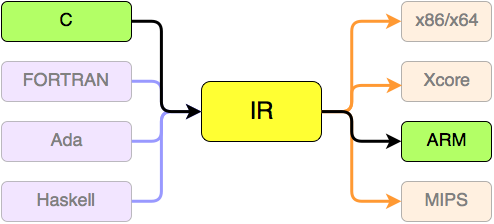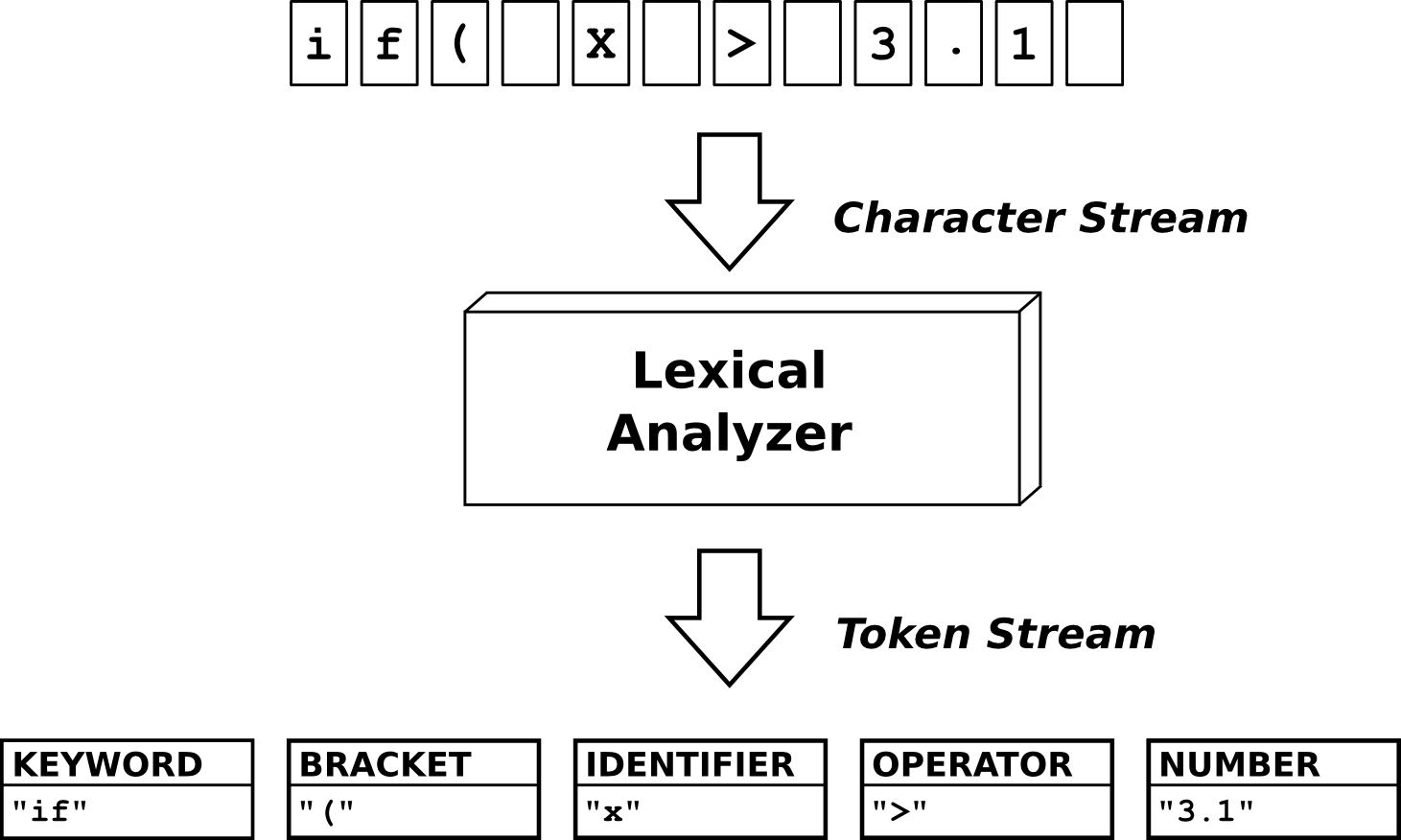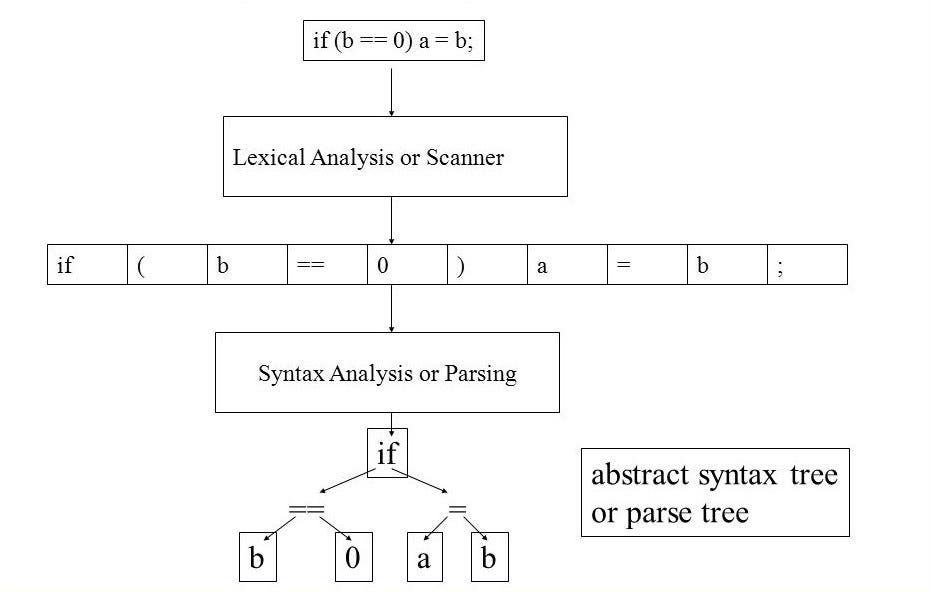# Writing your own programming language and compiler with PythonAfter studying compilers and programming languages, I felt like internet tutorials and guides are way to complex for beginners or are missing some important parts about these topics.

### Introduction

After studying compilers and programming languages, I felt like internet tutorials and guides are way to complex for beginners or are missing some important parts about these topics.

My goal with this post is to help people that are seeking a way to start developing their first programming language/compiler.

### Requirements

On this guide, I’ll be using PLY as lexer and parser, and LLVMlite as low level intermediate language to do code generation with optimizations (if you don’t know what I’m talking about, don’t worry, I’ll explain it later).

So, the requirements for this project are:

• Anaconda (way simpler to install LLVMlite through conda than pip)
• LLVMlite
``````\$ conda install --channel=numba llvmlite
``````
• RPLY (same as PLY but with a better API)
``````\$ conda install -c conda-forge rply
``````
• LLC (LLVM static compiler)
• GCC (or other linking tool)

### Getting Started

“Where to begin?”. This is the most common question when trying to create your programming language.

I’ll start by defining my own language. Let’s call it TOY, here’s a simple example of a TOY program:

``````var x;
x := 4 + 4 * 2;
if (x > 3) {
x := 2;
} else {
x := 5;
}
print(x);
``````

Although this example is really simple, it is not so easy to be implemented as a programming language. So let’s start with a simpler example:

``````print(4 + 4 - 2);
``````

But how do you formally describe a language grammar? It is way to hard to create all possible examples of a language to show everything it can do.

To do this, we do what is called an EBNF. It is a metalanguage to define with one document, all possible grammar structures of a language. You can find most programming languages EBNFs easily.

To understand better how a EBNF grammar works, I recommend reading this post.

### EBNF

Let’s create a EBNF that describes the minimal possible functionality of TOY, only a sum operation_._ It will describe the following example:

``````4 + 2;
``````

It’s EBNF can be described as:

``````expression = number, "+", number, ";";
number = digit+;
digit = [0-9];
``````

This example is way to simple to be useful as a programming language, so let’s add some functionalities. The first one, is to be able to add as many numbers as you want, and the second one, is to to be able to subtract numbers as well.

Here’s an example of our new programming language:

``````4 + 4 - 2;
``````

And it’s EBNF can be described as:

``````expression = number, { ("+"|"-"), number }, ";";
number = digit+;
digit = [0-9];
``````

And finally, adding print to our programming language:

``````print(4 + 4 - 2);
``````

We get to this EBNF:

``````program = "print", "(", expression, ")", ";";
expression = number, { ("+"|"-"), number } ;
number = digit+
digit = [0-9];
``````

Now that we’ve defined our grammar, how do we translate it to code? So we can validate and understand a program? And after that, how can we compile it to a binary executable?

### Compiler

A compiler is a program that turns a programming language into machine language or other languages. In this guide, I’m going to compile our programming language into LLVM IR and then into machine language.Using LLVM, it is possible to optimize your compilation without learning compiling optimization, and LLVM has a really good library to work with compilers.

Our compiler can be divided into three components:

• Lexer
• Parser
• Code Generator

For the Lexer and Parser we’ll be using RPLY, really similar to PLY: a Python library with lexical and parsing tools, but with a better API. And for the Code Generator, we’ll use LLVMlite, a Python library for binding LLVM components.

### Lexer

The first component of out compiler is the Lexer. It’s role is to take the program as input and divide it into Tokens.We use the minimal structures from out EBNF to define our tokens. For example, with the following input:

``````print(4 + 4 - 2);
``````

Out Lexer would divide this string into this list of tokens:

``````Token('PRINT', 'print')
Token('OPEN_PAREN', '(')
Token('NUMBER', '4')
Token('SUM', '+')
Token('NUMBER', '4')
Token('SUB', '-')
Token('NUMBER', '2')
Token('CLOSE_PAREN', ')')
Token('SEMI_COLON', ';')
``````

So, let’s start coding our compiler. First, create a file named `lexer.py`. We’ll define our tokens on this file. We’ll only use LexerGenerator class from RPLY to create our Lexer.

lexer.py

``````from rply import LexerGenerator

class Lexer():
def __init__(self):
self.lexer = LexerGenerator()

# Print
# Parenthesis
# Semi Colon
# Operators
# Number
# Ignore spaces
self.lexer.ignore('\s+')

def get_lexer(self):
return self.lexer.build()
``````

After this, create your main file named `main.py` . We’ll combine all three compiler components on this file.

main1.py

``````from lexer import Lexer

text_input = """
print(4 + 4 - 2);
"""

lexer = Lexer().get_lexer()
tokens = lexer.lex(text_input)

for token in tokens:
print(token)
``````

If you run `\$ python main.py` , the output of tokens will be the same as described above. You can change the name of your tokens if you want, but I recommend keeping the same to keep consistency with the Parser.

### Parser

The second component in our compiler is the Parser. It’s role is to do a syntax check of the program. It takes the list of tokens as input and create an AST as output. This concept is more complex than a list of tokens, so I highly recommend a little bit of research about Parsers and ASTs.To implement our parser, we’ll use the structure created with out EBNF as model. Luckly, RPLY’s parser uses a format really similar to the EBNF to create it’s parser, so it is really straightforward.

The most challenging is to attach the Parser with the AST, but when you get the idea, it becomes really mechanical.

First, create a new file named `ast.py` . It will contain all classes that are going to be called on the parser and create the AST.

ast1.py

``````class Number():
def __init__(self, value):
self.value = value

def eval(self):
return int(self.value)

class BinaryOp():
def __init__(self, left, right):
self.left = left
self.right = right

class Sum(BinaryOp):
def eval(self):
return self.left.eval() + self.right.eval()

class Sub(BinaryOp):
def eval(self):
return self.left.eval() - self.right.eval()

class Print():
def __init__(self, value):
self.value = value

def eval(self):
print(self.value.eval())
``````

Second, we need to create the parser. For that, we’ll use ParserGenerator from RPLY. Create a file name `parser.py:`
parser1.py

``````from rply import ParserGenerator
from ast import Number, Sum, Sub, Print

class Parser():
def __init__(self):
self.pg = ParserGenerator(
# A list of all token names accepted by the parser.
['NUMBER', 'PRINT', 'OPEN_PAREN', 'CLOSE_PAREN',
'SEMI_COLON', 'SUM', 'SUB']
)

def parse(self):
@self.pg.production('program : PRINT OPEN_PAREN expression CLOSE_PAREN SEMI_COLON')
def program(p):
return Print(p)

@self.pg.production('expression : expression SUM expression')
@self.pg.production('expression : expression SUB expression')
def expression(p):
left = p
right = p
operator = p
if operator.gettokentype() == 'SUM':
return Sum(left, right)
elif operator.gettokentype() == 'SUB':
return Sub(left, right)

@self.pg.production('expression : NUMBER')
def number(p):
return Number(p.value)

@self.pg.error
def error_handle(token):
raise ValueError(token)

def get_parser(self):
return self.pg.build()
``````

And finally, we’ll update our file `main.py` to combine Parser with Lexer.
main2.py

``````from lexer import Lexer
from parser import Parser

text_input = """
print(4 + 4 - 2);
"""

lexer = Lexer().get_lexer()
tokens = lexer.lex(text_input)

pg = Parser()
pg.parse()
parser = pg.get_parser()
parser.parse(tokens).eval()
``````

Now, if you run `\$ python main.py`, you’ll see the output being the result of `print(4 + 4 — 2)`, which is equal to printing 6.

With these two components, we have a functional compiler that interprets TOY language with Python. However, it still doesn’t create a machine language code and is not well optimized. To do this, we’ll enter the most complex part of the guide, code generation with LLVM.

### Code Generator

The third and last component of out compiler is the Code Generator. It’s role is to transform the AST created from the parser into machine language or an IR. In this case, it’s going to transform the AST into LLVM IR.

This component is the main reason whyI’m writing this post. There aren’t good guides on how to implement code generation with LLVM on Python.

LLVM can be really complex to understand, so if you wish to fully understand what is going on, I recommend reading LLVMlite docs.

LLVMlite doesn’t have a implementation to a `print` function, so you have to define your own.

So, to start, let’s create a file named `codegen.py` that will contain the class `CodeGen`. This class is responsible to configure LLVM and create and save the IR code. We also declare the Print function on it.
codegen.py

``````from llvmlite import ir, binding

class CodeGen():
def __init__(self):
self.binding = binding
self.binding.initialize()
self.binding.initialize_native_target()
self.binding.initialize_native_asmprinter()
self._config_llvm()
self._create_execution_engine()
self._declare_print_function()

def _config_llvm(self):
# Config LLVM
self.module = ir.Module(name=__file__)
self.module.triple = self.binding.get_default_triple()
func_type = ir.FunctionType(ir.VoidType(), [], False)
base_func = ir.Function(self.module, func_type, name="main")
block = base_func.append_basic_block(name="entry")
self.builder = ir.IRBuilder(block)

def _create_execution_engine(self):
"""
Create an ExecutionEngine suitable for JIT code generation on
the host CPU.  The engine is reusable for an arbitrary number of
modules.
"""
target = self.binding.Target.from_default_triple()
target_machine = target.create_target_machine()
# And an execution engine with an empty backing module
backing_mod = binding.parse_assembly("")
engine = binding.create_mcjit_compiler(backing_mod, target_machine)
self.engine = engine

def _declare_print_function(self):
# Declare Printf function
voidptr_ty = ir.IntType(8).as_pointer()
printf_ty = ir.FunctionType(ir.IntType(32), [voidptr_ty], var_arg=True)
printf = ir.Function(self.module, printf_ty, name="printf")
self.printf = printf

def _compile_ir(self):
"""
Compile the LLVM IR string with the given engine.
The compiled module object is returned.
"""
# Create a LLVM module object from the IR
self.builder.ret_void()
llvm_ir = str(self.module)
mod = self.binding.parse_assembly(llvm_ir)
mod.verify()
# Now add the module and make sure it is ready for execution
self.engine.finalize_object()
self.engine.run_static_constructors()
return mod

def create_ir(self):
self._compile_ir()

def save_ir(self, filename):
with open(filename, 'w') as output_file:
output_file.write(str(self.module))
``````

After this, let’s update our `main.py` file to call `CodeGen` methods:

*main3.py *

``````from lexer import Lexer
from parser import Parser
from codegen import CodeGen

fname = "input.toy"
with open(fname) as f:

lexer = Lexer().get_lexer()
tokens = lexer.lex(text_input)

codegen = CodeGen()

module = codegen.module
builder = codegen.builder
printf = codegen.printf

pg = Parser(module, builder, printf)
pg.parse()
parser = pg.get_parser()
parser.parse(tokens).eval()

codegen.create_ir()
codegen.save_ir("output.ll")
``````

As you can see, I removed the input program from this file and created a new file called `input.toy` to simulate a external program. It’s content is the same as the input described.

``````print(4 + 4 - 2);
``````

Another change that was made is passing `module`, `builder` and `printf` objects to the Parser. This was made so we could pass this objects to the AST, where the LLVM AST is created. So, we change `parser.py` to receive these objects and pass them to the AST.

``````from rply import ParserGenerator
from ast import Number, Sum, Sub, Print

class Parser():
def __init__(self, module, builder, printf):
self.pg = ParserGenerator(
# A list of all token names accepted by the parser.
['NUMBER', 'PRINT', 'OPEN_PAREN', 'CLOSE_PAREN',
'SEMI_COLON', 'SUM', 'SUB']
)
self.module = module
self.builder = builder
self.printf = printf

def parse(self):
@self.pg.production('program : PRINT OPEN_PAREN expression CLOSE_PAREN SEMI_COLON')
def program(p):
return Print(self.builder, self.module, self.printf, p)

@self.pg.production('expression : expression SUM expression')
@self.pg.production('expression : expression SUB expression')
def expression(p):
left = p
right = p
operator = p
if operator.gettokentype() == 'SUM':
return Sum(self.builder, self.module, left, right)
elif operator.gettokentype() == 'SUB':
return Sub(self.builder, self.module, left, right)

@self.pg.production('expression : NUMBER')
def number(p):
return Number(self.builder, self.module, p.value)

@self.pg.error
def error_handle(token):
raise ValueError(token)

def get_parser(self):
return self.pg.build()
``````

And finally, and most important, we change the `ast.py` to receive these objects and create the LLVM AST using LLVMlite methods.
ast2.py

``````from llvmlite import ir

class Number():
def __init__(self, builder, module, value):
self.builder = builder
self.module = module
self.value = value

def eval(self):
i = ir.Constant(ir.IntType(8), int(self.value))
return i

class BinaryOp():
def __init__(self, builder, module, left, right):
self.builder = builder
self.module = module
self.left = left
self.right = right

class Sum(BinaryOp):
def eval(self):
return i

class Sub(BinaryOp):
def eval(self):
i = self.builder.sub(self.left.eval(), self.right.eval())
return i

class Print():
def __init__(self, builder, module, printf, value):
self.builder = builder
self.module = module
self.printf = printf
self.value = value

def eval(self):
value = self.value.eval()

# Declare argument list
voidptr_ty = ir.IntType(8).as_pointer()
fmt = "%i \n\0"
c_fmt = ir.Constant(ir.ArrayType(ir.IntType(8), len(fmt)),
bytearray(fmt.encode("utf8")))
global_fmt = ir.GlobalVariable(self.module, c_fmt.type, name="fstr")
global_fmt.global_constant = True
global_fmt.initializer = c_fmt
fmt_arg = self.builder.bitcast(global_fmt, voidptr_ty)

# Call Print Function
self.builder.call(self.printf, [fmt_arg, value])
``````

With these changes, our compiler is ready to transform a TOY program into an LLVM IR file `output.ll`. To compile this `.ll` file into a executable, we’ll use LLC to create a object file `output.o`, and finally, GCC (you could use other linking programs) to create the final executable file.

``````\$ llc -filetype=obj output.ll
\$ gcc output.o -o output
``````

And you can finally run your executable file compiled from the initial program.

``````\$ ./output
``````

### Next Steps

After this guide, I hope you can understand an EBNF and the three basic concepts of a compiler. With this knowledge, you now can create your own programming language and write a optimized compiler to it with Python. I encourage you to go further and add new elements to your language and compiler, here are some ideas:

• Statements
• Variables
• New Binary Operators ( Multiplication, Division)
• Unary Operators
• If Statement
• While Stametement

Feel free to send me any compiler projects. I’ll be happy to help you with anything.

You can contact me at [email protected]

Hope you all enjoyed this post and got some love to programming languages and compilers!

You can see the final code on GitHub.